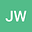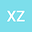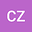loading page

Statistical Solutions for a Nonautonomous Modified Swift-Hohenberg Equation
•••Xiaoqian Zhang
Wenzhou University
Author ProfileCaidi Zhao
Wenzhou University
Author Profile## Abstract

We consider the nonautonomous modified Swift-Hohenberg equation $$u_t+\Delta^2u+2\Delta u+au+b|\nabla u|^2+u^3=g(t,x)$$ on a bounded smooth domain $\Omega\subset\R^n$ with $n\leqslant 3$. We show that, if $|b|<4$ and the external force $g$ satisfies some appropriate assumption, then the associated process has a unique pullback attractor in the Sobolev space $H_0^2(\Omega)$. Based on this existence, we further prove the existence of a family of invariant Borel probability measures and a statistical solution for this equation.

#### Peer review status:UNDER REVIEW

27 Apr 2021Submitted to Mathematical Methods in the Applied Sciences
28 Apr 2021Assigned to Editor
28 Apr 2021Submission Checks Completed
01 May 2021Reviewer(s) Assigned